# Flower bed

The park has a rectangular flower bed with dimensions of 3.2m and 1.5m. How many rose bushes will we plant in the flower bed if 20 dm2 are needed for one bush?

n =  24

### Step-by-step explanation:Did you find an error or inaccuracy? Feel free to write us. Thank you!Tips to related online calculators
Do you want to convert area units?

#### You need to know the following knowledge to solve this word math problem:

We encourage you to watch this tutorial video on this math problem:

## Related math problems and questions:

• SeedsThe field has a rectangular shape with dimensions of 128 m and 350 m. How many kg of seed are needed for sowing if the 1 m2 will consume 25 g of seeds?
• Rectangular flowerbedAround the rectangular flowerbed with dimensions of 5.25 m and 3.50 m, roses should be planted at the same distance from each other so that the roses are located in each corner of the flower bed and are consumed as little as possible. How far do we plant
• Flowerbed 2Around the square flower bed in a park is sidewalk 2 m wide. The area of ​​this sidewalk is 243 m2. What is the area of the flowerbed?
• Octagon from rectangleFrom tablecloth rectangular shape with dimensions of 4 dm and 8 dm we cuts down the corners in the shape of isosceles triangles. It thus formed an octagon with area 26 dm2. How many dm2 we cuts down?
• Room dimensions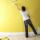Room dimensions are 5m and 3.5m. Room height is 2.85m. Paint the room (even with the ceiling). There will be 2 layers. Doors and windows have a total of 2.5 m2. One box of paint is enough for 6m2. How many boxes of paint are needed? How much do we pay if
• Pavilion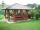The rectangular pavilion with dimensions 3.5 m and 2.75 m to be paved with square tiles of side 25 cm price of CZK 22 per 1 piece or rectangular tiles with sides of 20 cm and 15 cm in the price of CZK 11 per 1 pc. Which solution is cheaper (write its pric
• Square plate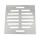The locksmith cut a square metal plate with a sidelong 6 dm into two identical rectangular openings with 2.5 dm and 2 dm dimensions. Calculate the remainder of the square plate.
• Tiles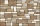The rectangular floor of the 6 x 1.8 m dimensions is to be covered with 50 cm square tiles. How many tiles will be needed?
• Two sides paintThe door has the shape of a rectangle with dimensions of 260cm and 170cm. How many cans of paint will be needed to paint this door if one can of paint cover 2m2 of the area? We paint the doors on both sides.
• Flooring a roomFind the cost of flooring a room 6.5 m by 5 m with square tiles of sides 25 cm at the rate of rupees 9.40 per tile.
• GardenThe rectangular garden has dimensions of 27 m and 30 m. Peter and Katka split it in a ratio of 4:5. How many square meters did Katkin measure part of the garden?
• Scale of planOn the plan of the village in the scale of 1: 1000 a rectangular garden is drawn. Its dimensions on the plan are 25mm and 28mm. Determine the area of the garden in ares.
• SquareCalculate the area of the square shape of the isosceles triangle with the arms 50m and the base 60m. How many tiles are used to pave the square if the area of one tile is 25 dm2?
• Wall painting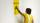The wall is 4 meters wide and 2 meters high. The window in the wall has dimensions of 2x1,8 meters. How many liters of color is needed to paint two-layer these walls, if the 1 m2 needs 1 liters of paint?
• Area of rectangleCalculate the rectangle area in square meters whose sides have dimensions a = 80dm and b = 160dm.
• Two walls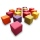Calculate the surface area of a cube in m2 if you know that the area of its two walls is 72 dm2.
• Pool tilesThe pool is 25m long, 10m wide, and 160cm deep. How many m2 of tiles will be needed on the walls and the pool? How many tiles are needed when 1 tile has a square shape with a 20cm side? How much does it cost when 1m2 of tiles costs 258 Kc?